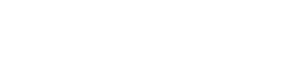# Phi Coefficient (Mean Square Contingency Coefficient)

Correlation Coefficients > Phi Coefficient

## What is the Phi Coefficient?

The Phi Coefficient is a measure of association between two binary variables (i.e. living/dead, black/white, success/failure). It is also called the Yule phi or Mean Square Contingency Coefficient and is used for contingency tables when:A simple contingency table. Image: Michigan Dept. of Agriculture

For a 2×2 contingency table where a, b, c, and d represent the observation frequencies (the cell count). The formula for phi is:Example: Find phi for the following contingency table:Solution: Insert the counts into the formula and solve.
Φ = ad – bc / √((a + b)(c + d)(a + c)(b + d))
Φ = 14*13 – 10*6 / √((14 + 10)(6 + 13)(14 + 6)(10 + 13))
Φ = 182 – 60 / √((24)(19)(20)(23))
Φ = 122/ √((24)(19)(20)(23))
Φ = 122/ 458
Φ = 0.266.

## Interpreting the Phi Coefficient

The phi coefficient is a symmetrical statistic, which means the independent variable and dependent variables are interchangeable.
The interpretation for the phi coefficient is similar to the Pearson Correlation Coefficient. The range is from -1 to 1, where:

• 0 is no relationship.
• 1 is a perfect positive relationship: most of your data falls along the diagonal cells.
• -1 is a perfect negative relationship: most of your data is not on the diagonal.

The Political Science Department at Quinnipiac University posted this useful list of the meaning of Pearson’s Correlation coefficients. The same rule of thumb can be used for the Phi coefficient. Note that these are “crude estimates” for interpreting strengths of relationships.

 r value = +.70 or higher Very strong positive relationship +.40 to +.69 Strong positive relationship +.30 to +.39 Moderate positive relationship +.20 to +.29 weak positive relationship +.01 to +.19 No or negligible relationship 0 No relationship -.01 to -.19 No or negligible relationship -.20 to -.29 weak negative relationship -.30 to -.39 Moderate negative relationship -.40 to -.69 Strong negative relationship -.70 or higher Very strong negative relationship

References:
Quinnipiac University. Maaning of Pearson’s. Retrieved Jun 20, 2016 from: http://faculty.quinnipiac.edu/libarts/polsci/Statistics.html
Yule, G. U. (1912). J. R. Statist. Soc., 75,
576–642. (On the methods of measuring the
association between two variables. The first
identification of the phi-coefficient.)

CITE THIS AS:
Stephanie Glen. "Phi Coefficient (Mean Square Contingency Coefficient)" From StatisticsHowTo.com: Elementary Statistics for the rest of us! https://www.statisticshowto.com/phi-coefficient-mean-square-contingency-coefficient/
---------------------------------------------------------------------------Need help with a homework or test question? With Chegg Study, you can get step-by-step solutions to your questions from an expert in the field. Your first 30 minutes with a Chegg tutor is free!